Download Presentation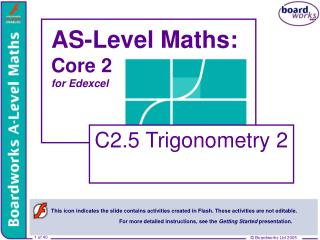AS-Level Maths: Core 2 for Edexcel

# AS-Level Maths: Core 2 for Edexcel - PowerPoint PPT Presentation

AS-Level Maths: Core 2 for Edexcel. C2.5 Trigonometry 2. This icon indicates the slide contains activities created in Flash. These activities are not editable. For more detailed instructions, see the Getting Started presentation. 1 of 40. © Boardworks Ltd 2005. The sine rule.I am the owner, or an agent authorized to act on behalf of the owner, of the copyrighted work described.
Download Presentation## AS-Level Maths: Core 2 for Edexcel

An Image/Link below is provided (as is) to download presentation

Download Policy: Content on the Website is provided to you AS IS for your information and personal use and may not be sold / licensed / shared on other websites without getting consent from its author.While downloading, if for some reason you are not able to download a presentation, the publisher may have deleted the file from their server.

- - - - - - - - - - - - - - - - - - - - - - - - - - E N D - - - - - - - - - - - - - - - - - - - - - - - - - -
Presentation Transcript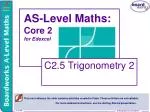### AS-Level Maths:Core 2for Edexcel

C2.5 Trigonometry 2

This icon indicates the slide contains activities created in Flash. These activities are not editable.

For more detailed instructions, see the Getting Started presentation.

1 of 40

© Boardworks Ltd 2005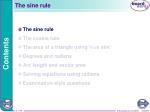The sine rule
• The sine rule
• The cosine rule
• The area of a triangle using ½ ab sinC
• Degrees and radians
• Arc length and sector area
• Solving equations using radians
• Examination-style questions

Contents

2 of 40

© Boardworks Ltd 2005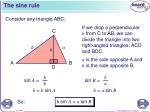The sine rule

h

h

a

b

Consider any triangle ABC:

If we drop a perpendicular h from C to AB, we can divide the triangle into two right-angled triangles; ACD and BDC.

C

b

a

h

a is the side opposite A and b is the side opposite B.

A

B

D

sin B =

sin A =

h = a sin B

h = b sin A

b sin A = a sin B

So: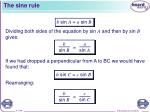The sine rule

a

c

b

b

=

=

sin A

sin C

sin B

sin B

b sin A = a sin B

Dividing both sides of the equation by sin A and then by sin B

gives:

If we had dropped a perpendicular from A to BC we would have found that:

b sin C = c sin B

Rearranging: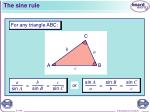The sine rule

C

b

a

A

B

c

b

c

a

sin A

sin B

sin C

=

=

=

=

a

b

c

sin B

sin C

sin A

For any triangle ABC:

or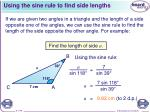Using the sine rule to find side lengths

B

39°

7

a

=

a

sin 39°

sin 118°

118°

7 sin 118°

a

=

C

A

7 cm

sin 39°

If we are given two angles in a triangle and the length of a side opposite one of the angles, we can use the sine rule to find the length of the side opposite the other angle. For example:

Find the length of side a.

Using the sine rule:

a = 9.82cm (to 2 d.p.)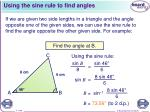Using the sine rule to find angles

C

sin 46°

sin B

=

8 cm

8

6

6 cm

8 sin 46°

sin B

=

6

8 sin 46°

46°

A

B

sin–1

B=

6

If we are given two side lengths in a triangle and the angle opposite one of the given sides, we can use the sine rule to find the angle opposite the other given side. For example:

Find the angle at B.

Using the sine rule:

B = 73.56° (to 2 d.p.)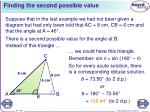Finding the second possible value

C

8 cm

6 cm

6 cm

46°

46°

A

B

B

Suppose that in the last examplewe had not been given a diagram but had only been told that AC = 8 cm, CB = 6 cm and that the angle at A = 46°.

There is a second possible value for the angle at B.

Instead of this triangle …

… we could have this triangle.

Remember: sin θ= sin (180° –θ)

So for every acute solution, there is a corresponding obtuse solution.

B = 73.56° (to 2 d.p.)

or

B = 180° – 73.56°

= 106.44° (to 2 d.p.)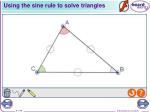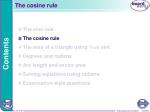The cosine rule
• The sine rule
• The cosine rule
• The area of a triangle using ½ ab sinC
• Degrees and radians
• Arc length and sector area
• Solving equations using radians
• Examination-style questions

Contents

10 of 40

© Boardworks Ltd 2005The cosine rule

Consider any triangle ABC:

If we drop a perpendicular h from C to AB, we can divide the triangle into two right-angled triangles; ACD and BDC.

C

b

a

h

a is the side opposite A and b is the side opposite B.

A

B

x

D

c – x

c is the side opposite C. If AD = x, then the length BD can be written as c – x.The cosine rule

1

2

cos A =

x

b

Substituting and gives:

1

2

Using Pythagoras’ theorem in triangle ACD:

C

b2 = x2 +h2

b

a

h

Also:

A

B

x

D

c – x

x = b cos A

In triangle BCD:

a2 = (c – x)2 + h2

a2 = c2 – 2cx+ x2 + h2

a2 = c2 – 2cx + x2 + h2

This is the cosine rule.

a2 = c2 – 2cbcos A+ b2

a2 = b2 + c2 – 2bc cos AThe cosine rule

A

c

b

b2 + c2 – a2

B

C

cos A =

a

2bc

For any triangle ABC:

a2 = b2 + c2 – 2bc cos A

orUsing the cosine rule to find side lengths

B

a

4 cm

48°

A

C

7 cm

If we are given the length of two sides in a triangle and the size of the angle between them, we can use the cosine rule to find the length of the other side. For example:

Find the length of side a.

a2 = b2 + c2 – 2bc cos A

a2 = 72 + 42 – (2× 7 × 4 × cos 48°)

a2 = 27.53 (to 2 d.p.)

a = 5.25 cm (to 2 d.p.)Using the cosine rule to find angles

B

b2 + c2 – a2

cos A =

2bc

8 cm

42 + 62 – 82

cos A =

6 cm

2 × 4 × 6

C

A

4 cm

If we are given the lengths of all three sides in a triangle, we can use the cosine rule to find the size of any one of the angles in the triangle. For example:

Find the size of the angle at A.

This is negative so A must be obtuse.

cos A = –0.25

A = cos–1 –0.25

A = 104.48° (to 2 d.p.)The area of a triangle using ½ ab sinC
• The sine rule
• The cosine rule
• The area of a triangle using ½ ab sin C
• Degrees and radians
• Arc length and sector area
• Solving equations using radians
• Examination-style questions

Contents

17 of 40

© Boardworks Ltd 2005The area of a triangle

A

4 cm

47°

C

B

= sin 47°

7 cm

h

4

The area of a triangle is given by ½× base × height.

Suppose that instead of the height of a triangle, we are given the base, one of the sides and the included angle. For example:

What is the area of triangle ABC?

We can find the height h using the sine ratio.

h

h = 4 sin 47°

Area of triangle ABC = ½ × base × height

= ½ × 7 × 4 sin 47°

= 10.2 cm2(to 1 d.p.)The area of a triangle using ½ ab sin C

1

Area of triangle ABC = ab sin C

2

In general, the area of a triangle is equal to half the product of two of the sides and the sine of the included angle.

A

c

b

B

C

a• The sine rule
• The cosine rule
• The area of a triangle using ½ ab sin C
• Degrees and radians
• Arc length and sector area
• Solving equations using radians
• Examination-style questions

Contents

21 of 40

© Boardworks Ltd 2005Measuring angles in degrees

The system of using degrees to measure angles, where 1° is equal to of a full turn, is attributed to the ancient Babylonians.

For example, of a full turn is equal to 160°.

An angle is a measure of rotation.

The use of the number 360 is thought to originate from the approximate number of days in a year.

360 is also a number that has a high number of factors and so many fractions of a full turn can be written as a whole number of degrees.Measuring angles in radians

r

r

O

r

So: 1 rad =

In many mathematical and scientific applications, particularly in calculus, it is more appropriate to measure angles in radians.

A full turn is divided into 2π radians.

Remember that the circumference of a circle of radius r is equal to 2πr.

One radian is therefore equal to the angle subtended by an arc of length r.

1 radian can be written as 1 rad or 1c.

2π rad = 360°Converting radians to degrees

Or:

π rad = 180°

If the angle is not given in terms of π, when using a calculator for example, it can be converted to degrees by multiplying by

For example:

We can convert radians to degrees using:

2π rad = 360°

Radians are usually expressed as fractions or multiples of πso, for example:Converting degrees to radians

To convert degrees to radians we multiply by .

For example:

10

3

9

Sometimes angles are required to a given number of decimal places, rather than as multiples of π,for example:

Note that when radians are written in terms of π the units rad or c are not normally needed.Arc length and sector area
• The sine rule
• The cosine rule
• The area of a triangle using ½ ab sin C
• Degrees and radians
• Arc length and sector area
• Solving equations using radians
• Examination-style questions

Contents

27 of 40

© Boardworks Ltd 2005Using radians to measure arc length

A

r

θ

B

O

r

Suppose an arc AB of a circle of radius r subtends an angle of θ radians at the centre.

If the angle at the centre is 1 radian then the length of the arc is r.

If the angle at the centre is 2 radians then the length of the arc is 2r.

If the angle at the centre is 0.3 radians then the length of the arc is 0.3r.

In general:

Length of arc AB = θr

where θis measured in radians.

When θis measured in degrees the length of AB isFinding the area of a sector

Again suppose an arc AB subtends an angle of θradians at the centre O of a circle.

A

So the area of the sector AOB is

of the area of the full circle.

r

θ

B

O

r

 Area of sector AOB =

Area of sector AOB = r2θ

In general:

We can also find the area of a sector using radians.

The angle at the centre of a full circle is 2π radians.

where θis measured in radians.

When θis measured in degrees the area of AOB isFinding chord length and sector area

A chord AB subtends an angle of radians at the centre O of a circle of radius 9 cm. Find in terms of π:

a) the length of the arc AB.

b) the area of the sector AOB.

A

B

O

9 cm

b) area of sector AOB = r2θ

a) length of arc AB = θr

= 6π cm

= 27π cm2Finding the area of a segment

A

45°

B

O

5 cm

The formula for the area of a sector can be combined with the formula for the area of a triangle to find the area of a segment. For example:

A chord AB divides a circle of radius 5 cm into two segments. If AB subtends an angle of 45° at the centre of the circle, find the area of the minor segment to 3 significant figures.

Let’s call the area of sector AOB AS and the area of triangle AOB AT.Finding the area of a segment

Now:

Area of the minor segment = AS – AT

= 9.8174… – 8.8388…

= 0.979 cm2 (to 3 sig. figs.)

In general, the area of a segment of a circle of radius r is:

where θis measured in radians.Solving equations using radians
• The sine rule
• The cosine rule
• The area of a triangle using ½ ab sin C
• Degrees and radians
• Arc length and sector area
• Solving equations using radians
• Examination-style questions

Contents

33 of 40

© Boardworks Ltd 2005Solving equations using radians

Solve 4 cos 2θ= 2 for .

If the range for the solution set of a trigonometric equation is given in radians then the solution must also be given in radians. For example:

4 cos 2θ= 2

So: cos 2θ= 0.5

Changing the range to match the multiple angle:

–π≤ 2θ≤ π

If we now let x = 2θwe can solve cos x = 0.5 in the range –π≤ x ≤ π.Solving equations using radians

The principal solution of cos x = 0.5 is

if x =

θ =

This is the complete solution set in the range

Remember that cos is an even function and so, in general, cos (–θ) = cos θ.

 the second solution for x in the range –π≤ x ≤ π is x =

But x = 2θ, so:Examination-style questions
• The sine rule
• The cosine rule
• The area of a triangle using ½ ab sin C
• Degrees and radians
• Arc length and sector area
• Solving equations using radians
• Examination-style questions

Contents

36 of 40

© Boardworks Ltd 2005Examination-style question 1

sin 50°

sin C

=

7

6

7 sin 50°

sin B

=

6

7 sin 50°

sin–1

B=

6

• In the triangle ABC, AB = 7 cm, BC = 6 cm and = 50°.
• Calculate the two possible sizes of in degrees to two decimal places.
• Given that is obtuse, calculate the area of triangle ABC to two decimal places.

a) Using the sine rule:

B = 63.34°or 116.66° (to 2 d.p.)Examination-style question 1

Area of triangle ABC = × 6 × 7 × sin 13.34°

b) Area of triangle ABC = ac sin B

where a = 6 cm, c = 7 cm and B = (180 – 50 – 116.66)° = 13.34°

= 4.85 cm2 (to 2 d.p.)Examination-style question 2

A

B

6 cm

θ

O

10 cm

D

C

In the following diagram AC is an arc of a circle with centre O and radius 10 cm and BD is an arc of a circle with centre O and radius 6 cm.

• Find an expression for the area of the shaded region in terms of θ.
• Given that the shaded region is 25.6 cm2 find the value of θ.
• Calculate the perimeter of the shaded region.Examination-style question 2

Area of sector BOD = × 62 × θ

b)

32θ = 25.6

a) Area of sector AOC = × 102 × θ

= 50θ

= 18θ

Area of shaded region = 50θ – 18θ = 32θ

θ = 25.6 ÷ 32

θ = 0.8 radians

c) Perimeter of the shaded region

= length of arc AC + length of arc BD + AB + CD

= (10 × 0.8) + (6 × 0.8) + 8

= 20.8 cm